# Pandas dataframe, German vocabulary – select words by matching a few 3-char-grams – I

Words or strings can be segmented into so called „n-character-grams“ or „n-char-grams„. A n-char-gram is a defined sequence of „n“ letters, i.e. a special string of length „n“. Such a defined letter sequence – if short enough – can be found at various positions within many words of a vocabulary. Words or technically speaking „strings“ can e.g. be thought of being composed of a sequence of defined „2-char-grams“ or „3-char-grams“. „n-char-grams“ are useful for text-analysis and/or machine-learning methods applied to texts.

Let us assume you have a string representing a test word – but unfortunately with one or two wrong characters or two transposed characters at certain positions inside it. You may nevertheless want to find words in a German vocabulary which match most of the correct letters. One naive approach could be to compare the characters of the string position-wise with corresponding characters of all words in the vocabulary and then pick the word with most matches. As you neither can trust the first character nor the last character you quickly understand that a quick and efficient way of raising the probability to find reasonable fitting words requires to compare not only single letters but also bunches of them, i.e. sub-strings of sequential letters or „n-char-grams“.

This defines the problem of comparing n-char-grams at certain positions inside string „tokens“ extracted from unknown texts with n-char-grams of words in a vocabulary. I call a „token“ an unchecked distinct letter sequence, i.e. a string, identified by some „Tokenizer“-algorithm, which was applied to a text. A Tokenizer typically identifies word-separator characters to do his job. A „token“ might or might not be regular word of a language.

This mini-series looks a bit at using „3-character-grams“ of words in a German vocabulary residing in a Pandas dataframe. Providing and using 3-grams of a huge vocabulary in a suitable form as input for Python functions working on a Pandas dataframe can, however, be a costly business:

• RAM: First of all Pandas dataframes containing strings in most of the columns require memory. Using the dtype „category“ helps a lot to limit the memory consumption for a dataframe comprising all 3-char-grams of a reasonable vocabulary with some million words. See my last post on this topic.
• CPU-time: Another critical aspect is the CPU-time required to determine all dataframe rows, i.e. vocabulary words, which contain some given 3-char-grams at defined positions.
• It is not at all clear how many 3-char-grams are required to narrow down the spectrum of fitting words (of the vocabulary) for a given string to a small amount which can be handled by further detailed analysis modules.

In this article I, therefore, look at „queries“ on a Pandas dataframe containing vocabulary words plus their 3-char-grams at defined positions inside the words. Each column contains 3-char-grams at a defined position in the word strings. Our queries apply conditions to multiple selected columns. I first discuss how 3-char-grams split the vocabulary into groups. I present some graphs of how the number of words for such 3-char-gram based groups vary with 3-gram-position. Then the question how many 3-char-grams at different positions allow for an identification of a reasonably small bunch of fitting words in the vocabulary will be answered by some elementary experiments. We also look at CPU-times required for related queries and I discuss some elementary optimization steps. An eventual short turn to multiprocessing reveals that we, indeed, can gain a bit of performance.

As a basis for my investigations I use a „vocabulary“ based on the work of Torsten Brischalle. See
from http://www.aaabbb.de/WordList/WordList.php. I have supplemented his word-list by words with different writings for Umlauts. The word list contains around 2.8 million German words. Regarding the positional shift of the 3-char-grams of a word against each other I use the term „stride“ as explained in my last post
Pandas and 3-char-grams of a vocabulary – reduce memory consumption by datatype „category“.
In addition I use some „padding“ and fill up 3-char-grams at and beyond word boundaries with special characters (see the named post for it). In some plots I abbreviated „3-char-grams“ to „3-grams“.

# Why do I care about CPU-time on Pandas dataframes with 3-char-grams?

CPU-time is important if you want to correct misspelled words in huge bunches of texts with the help of 3-char-gram segmentation. Misspelled words are not only the result of wrong writing, but also of bad scans of old and unclear texts. I have a collection of over 200,000 such scans of German texts. The application of the Keras Tokenizer produced around 1.9 million string tokens.

Around 50% of the most frequent 100.000 tokens in my scanned texts appear to have „errors“ as they are no members of the (limited) vocabulary. The following plot shows the percentage of hits in the vocabulary against the absolute number of the most frequent words within the text collection: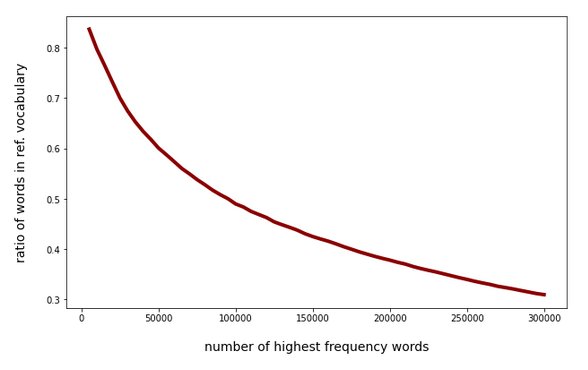The „errors“ contain a variety of (partially legitimate) compound words outside the vocabulary, but there are also wrong letters at different positions and omitted letters due to a bad OCR-quality of the scans. Correcting at least some of the simple errors (as one or two wrong characters) could improve the quality of the scan results significantly. To perform an analysis based on 3-char-grams we have to compare tenths up to hundreds of thousands tokens with some million vocabulary words. CPU-time matters – especially when using Pandas as a kind of database.

As the capabilities of my Linux workstation are limited I was interested in whether an analysis of 100,000 misspelled words based on comparisons of 3-char-grams is within reach for lets say a 100,000 tokens on a reasonably equipped PC.

# Major Objective: Reduce the amount of vocabulary words matching a few 3-char-grams at different string positions to a minimum

The analysis of possible errors of a scanned word is more difficult than one may think. The errors may be of different nature and may have different consequences for the length and structure of the resulting error-containing word in comparison with the originally intended word. Different error types may appear in combination and the consequences may interfere within a word (or identified token).

What you want to do is to find words in the vocabulary which are comparable to your token – at least in some major parts. The list of such words would be those which with some probability might contain the originally intended word. Then you might apply a detailed and error specific analysis to this bunch of interesting words. Such an analysis may be complemented by an additional analysis on (embedded) word-vector spaces created by ML-trained neural networks to predict words at the end of a sequence of other words. A detailed analysis on a list of words and their character composition in comparison to a token may be CU-time intensive in itself as it typically comprises string operations.

In addition it is required to do the job
a bit differently for certain error types and you also have to make some assumptions regarding the error’s impact on the word-length. But even under simplifying assumptions regarding the number of wrong letters and the correct total amount of letters in a token, you are confronted with a basic problem of error-correction:

You do not know where exactly a mistake may have occurred during scanning or wrong writing.

As a direct consequence you may have to compare 3-char-grams at various positions within the token with corresponding 3-char-grams of vocabulary words. But more queries mean more CPU-time ….

In any case one major objective must be to quickly reduce the amount of words of the vocabulary which you want to use in the detailed error analysis down to a minimum below 10 words with only a few Pandas queries. Therefore, two points are of interest here:

• How does the number of 3-char-grams for vocabulary words vary with the position?
• How many correct 3-char-grams define a word in the vocabulary on average?

The two aspects may, of course, be intertwined.

# Structure of the Pandas dataframe containing the vocabulary and its 3-char-grams

The image below displays the basic structure of the vocabulary I use in a Pandas dataframe (called „dfw_uml“):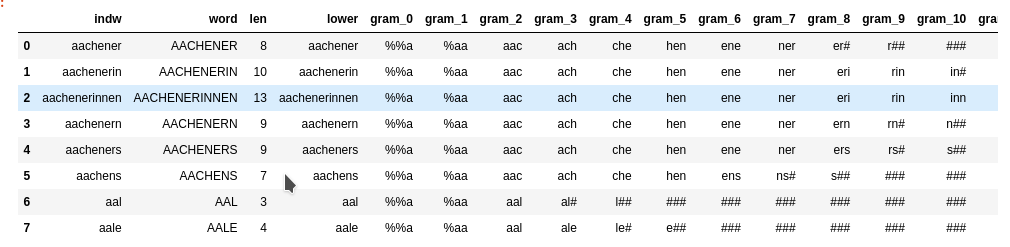The column „len“ contains the length of a word. The column „indw“ is identical to „lower“. „indw“ allows for a quick change of the index from integers to the word itself. Each column with „3-char-gram“ in the title corresponds to a defined position of 3-char-grams.

The stride between adjacent 3-char-grams is obviously 1. I used a „left-padding“ of 2. This means that the first 3-char-grams were supplemented by the artificial letter „%“ to the left. The first 3-char-gram with all letters residing within the word is called „gram_2“ in my case – with its leftmost letter being at position 0 of the word-string and the rightmost letter at position 2. On the right-most side of the word we use the letter „#“ to create 3-char-grams reaching outside the word boundary. You see that we get many „###“ 3-char-grams for short words at the right side of the dataframe.

Below I actually use two dataframes: one with 21 3-char-grams up to position 21 and another one with (55) 3-char-grams up to position 55.

# Variation of the number of vocabulary words against their length

With growing word-length there are more 3-char-grams to look at. Therefore we should have an idea about the distribution of the number of words with respect to word-length. The following plot shows how many different words we find with growing word-length in our vocabulary: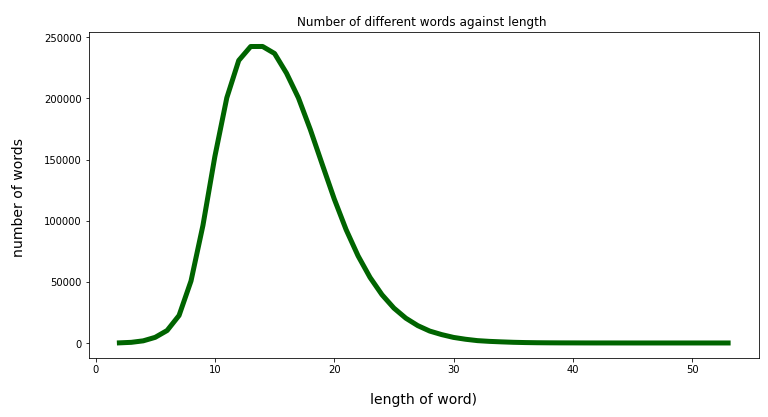The Python code for the plot above is :

```x1 = []
y1 = []
col_name = 'len'
df_col_grp_len = dfw_uml.groupby(col_name)['indw'].count()
d_len_voc = df_col_grp_len.to_dict()
#print (df_col_grp_len)
#print(d_len_voc)

len_d = len(d_len_voc)
for key,value in d_len_voc.items():
x1.append(key)
y1.append(value)

fig_size = plt.rcParams["figure.figsize"]
fig_size = 12
fig_size = 6
plt.plot(x1,y1, color='darkgreen', linewidth=5)
#plt.xticks(x)
plt.
plt.ylabel("number of words ", fontsize=14, labelpad=18)
plt.title("Number of different words against length ")
plt.show()
```

So, the word-length interval between 2 and 30 covers most of the words. This is consistent with the Pandas information provided by Pandas‘ „describe()“-function applied to column „len“: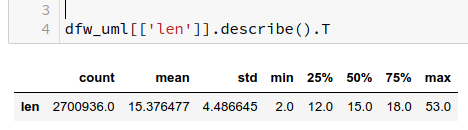# How does the number of different 3-char-grams vary with the 3-char-gram position?

Technically a 3-char-gram can be called „unique“ if it has a specific letter-sequence at a specific defined position. So would call the 3-char-grams „ena“ at position 5 and „ena“ at position 12 unique despite their matching sequence of letters.

There is only a limited amount of different 3-char-gram at a given position within the words of a given vocabulary.
Each 3-char-gram column of our dataframe can thus be divided into multiple „categories“ or groups of words containing the same specific 3-char-gram at the position associated with the column. A priori t was not at all clear to me how many vocabulary words we would typically find for a given 3-char-gram at a defined position. I wanted an overview. So let us first look at the number of different 3-char-grams against position.

So how does the distribution of the number of unique 3-char-grams against position look like?

To answer this question we use the Pandas function nunique() in the following way:

```# Determine number of unique values in columns )(i.e. against 3-char-gram position)
# ~~~~~~~~~~~~~~~~~~~~~~~~~~~~~~~~~~~~~~~~~~~~~
unique_vals = dfw_uml.nunique()
luv = len(unique_vals)
print(unique_vals)
```

and get

```.....
.....
gram_0          29
gram_1         459
gram_2        3068
gram_3        4797
gram_4        8076
gram_5        8687
gram_6        8743
gram_7        8839
gram_8        8732
gram_9        8625
gram_10       8544
gram_11       8249
gram_12       7829
gram_13       7465
gram_14       7047
gram_15       6700
gram_16       6292
gram_17       5821
gram_18       5413
gram_19       4944
gram_20       4452
gram_21       3989
```

Already in my last post we saw that the given different 3-char-grams at a defined position divide the vocabulary into a relatively small amount of groups. For my vocabulary with 2.8 million words the maximum number of different 3-char-grams is around 8,800 at position 7 (for a stride of 1). 8,800 is relatively small compared to the total number of 2.7 million words.

Above I looked at the 3-char-grams at the first 21 positions (including left-padding 3-char-grams). We can get a plot by applying the the following code

```# Plot for the distribution of categories (i.e. different 3-char-grams) against position
# **************************************
li_x = []
li_y = []
sum = 0

for i in range(0, luv-4):
li_x.append(i)
name = 'gram_' + str(i)
n_diff_grams = unique_vals[name]
li_y.append(n_diff_grams)
sum += n_diff_grams
print(sum)

fig_size = plt.rcParams["figure.figsize"]
fig_size = 12
fig_size = 6
plt.plot(li_x,li_y, color='darkblue', linewidth=5)
plt.xlim(1, 22)
plt.xticks(li_x)
plt.xlabel("3-gram position (3rd character)", fontsize=14, labelpad=18)
plt.ylabel("number of different 3-grams", fontsize=14, labelpad=18)
plt.show()
```

The plot is: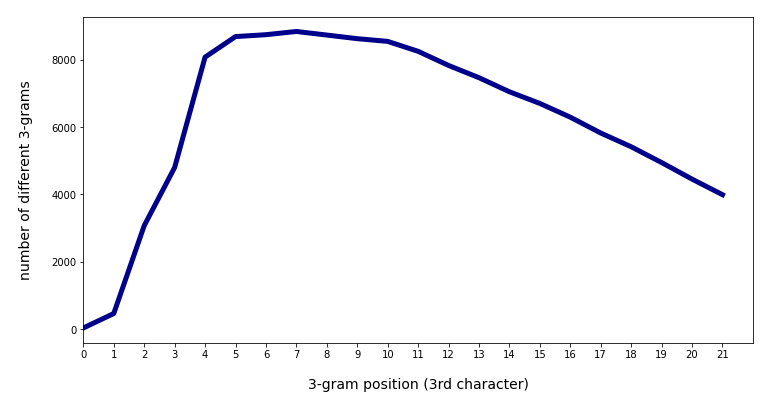We see a sharp rise of the number of different 3-char-grams with position 2 (i.e. with the 1st real character of the word) and a systematic decline after position 11. The total sum of all unique 3-char-grams over all positions 136,800 for positions up to 21. (The number includes padding-left and padding-right 3-char-grams).

When we extend the number of positions of 3-char-grams from 0 to 55 we get: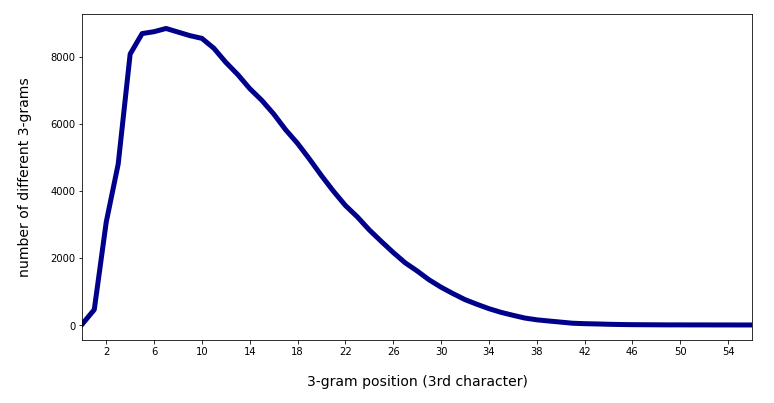The total sum of unique 3-char-grams then becomes 161,259.

# Maximum number of words per unique 3-char-gram with position

In a very similar way we can get the maximum number of rows, i.e. of different vocabulary words, appearing for a specific 3-char-gram at a certain position. This specific 3-char-gram defines the largest category or word group at the defined position. The following code creates a plot for the variation of this maximum against the 3-char-gram-position:

```# Determine max number of different rows per category
# ***********************************************
x = []
y = []
i_min = 0; i_max = 56
for j in range(i_min, i_max):
col_name = 'gram_' + str(j)
maxel = dfw_uml.groupby(col_name)['indw'].count().max()
x.append(j)
y.append(maxel)

fig_size = plt.rcParams["figure.figsize"]
fig_size = 12
fig_size = 6
plt.plot(x,y, color='darkred', linewidth=5)
plt.xticks(x)
plt.xlabel("3-gram position (3rd character)", fontsize=14, labelpad=18)
plt.ylabel("max number of words per 3-gram", fontsize=14, labelpad=18)
plt.show()
```

The result is: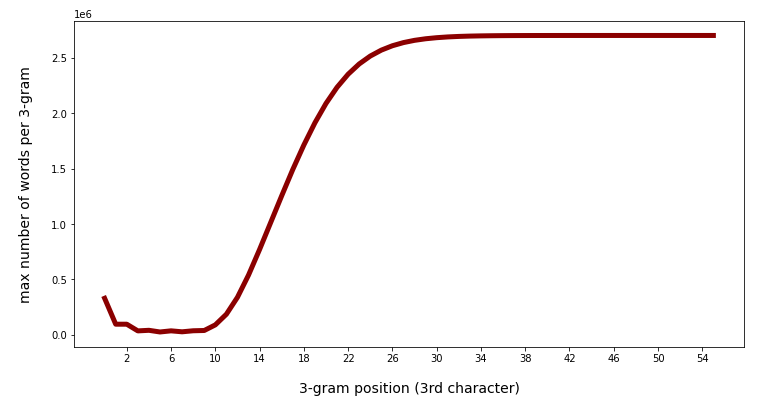The fact that there are less and less words with growing length in the vocabulary explains the growing maximum number of words for 3-char-grams at a defined late position. The maximum there corresponds to words for the artificial 3-char-gram „###“. Also the left-padding 3-char-grams have many fitting words.

Consistent to the number of different categories we get relatively small numbers between positions 3 and 9: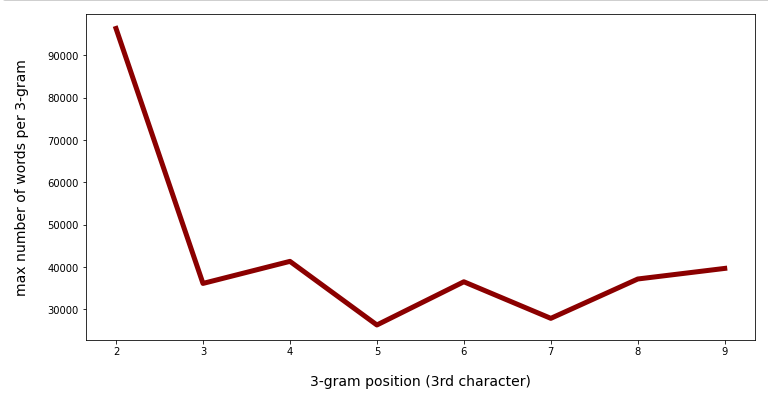Note that above we looked at the maximum, only. The various 3-char-grams defined at a certain position may have very different numbers of words being consistent with the 3-char-gram.

# Mean number of words with 3-char-gram position and variation at a certain position

Another view at the number of words per unique 3-char-gram is given by the average number of words for the 3-char-grams with position. The following graphs were produced by replacing the max()-function in the code above by the mean()-function:

Mean number of words per 3-char-gram category against positions 0 to 55: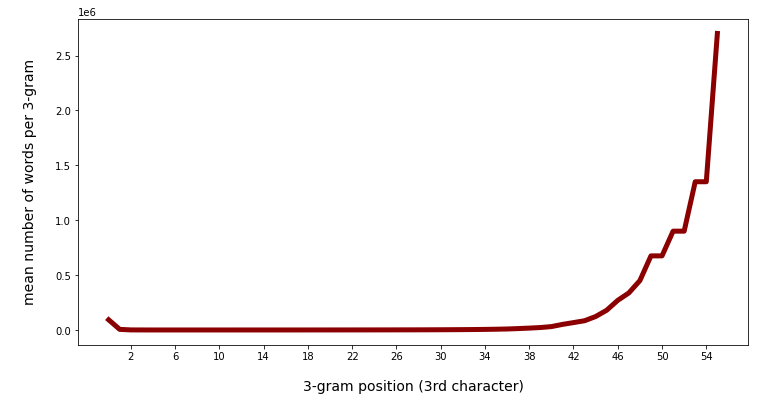Mean number of words per 3-char-gram category against positions 0 to 45: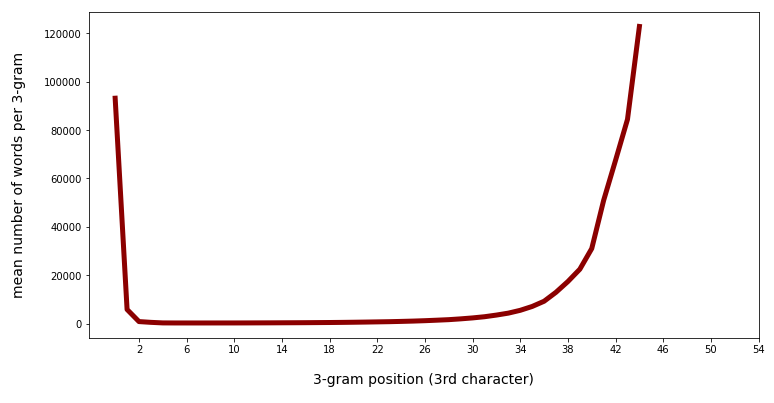We see that there is a significant slope after position 40. Going down to lower positions we see a more modest variation.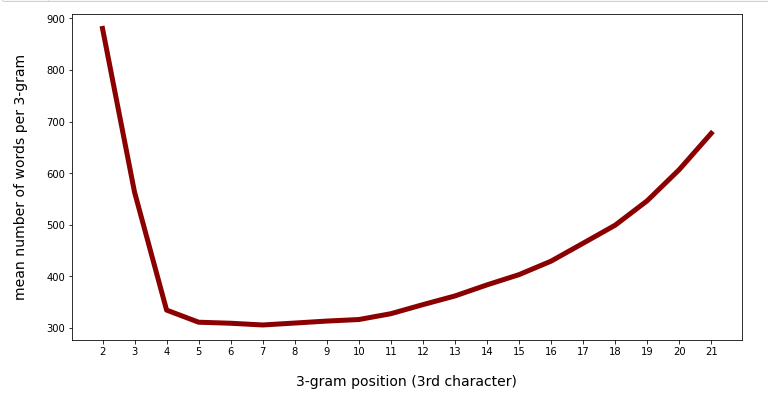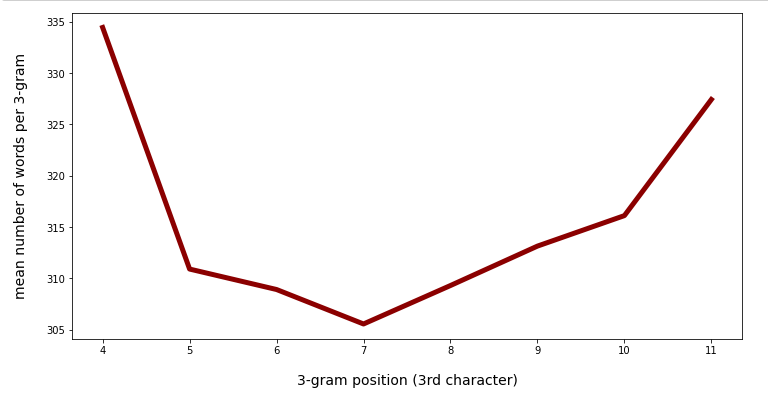There is some variation, but the total numbers are much smaller than the maximum numbers. This means that there is only a relatively small number of 3-char-grams which produce real big numbers.

This can also be seen from the following plots where I have ordered the 3-char-grams according to the rising number of matching words for the 3-char-grams at position 5 and at position 10: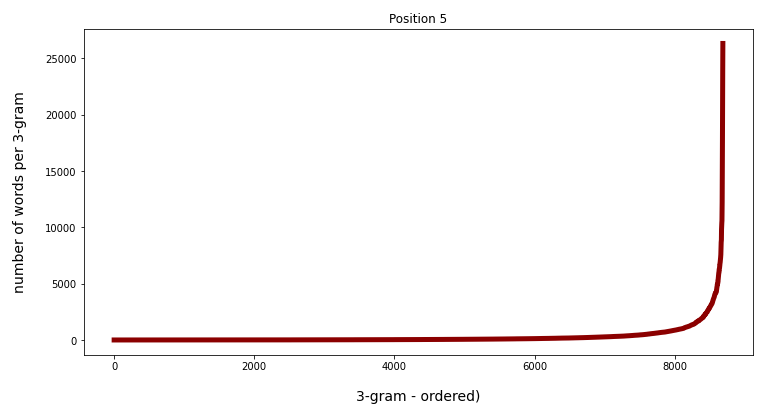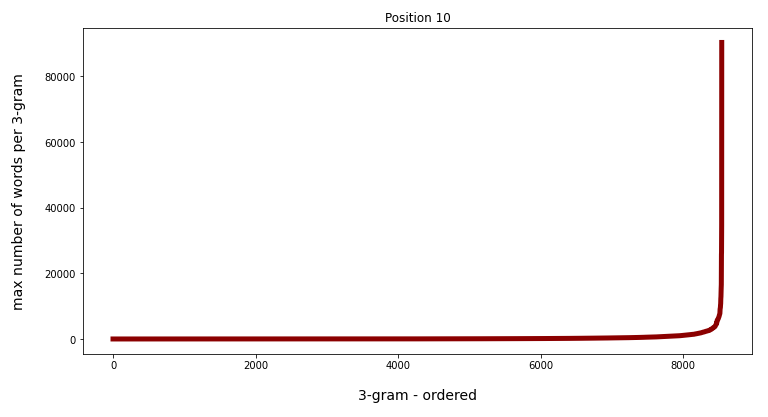Watch the different y-scales! When we limit the number of ordered grams to 8000 the variation is much more comparable: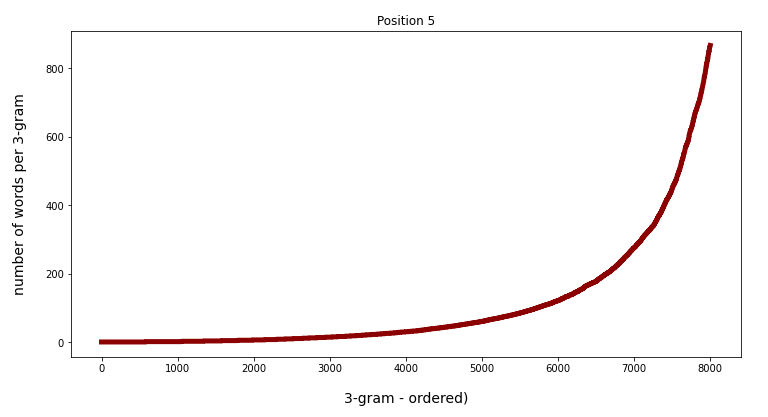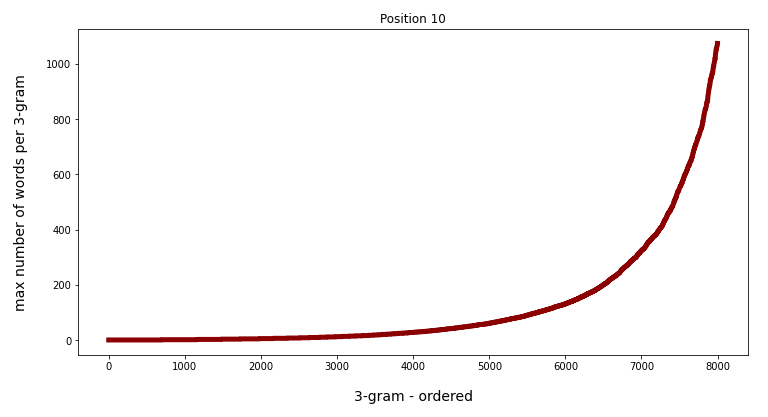# Conclusion

A quick overview over a vocabulary with the help of Pandas functions shows that the maximum and the mean number of matching words for 3-char-grams at defined positions inside the vocabulary words vary strongly with position and thereby also with word-length.

In the position range from 4 to 11 the mean number of words per unique 3-char-gram is pretty small – around 320. In the position range between 4 and 30 (covering most of the words) the mean number of different words per 3-char-gram is still below 1000.

This gives us some hope for reducing the number of words matching a few 3-char-grams at different positions down to numbers we can handle when applying a detailed analysis. The reason is that we then are interested in the intersection of multiple matching word-groups at the different positions. Respective queries, hit rates and CPU-Times are the topic of the next article:

Pandas dataframe, German vocabulary – select words by matching a few 3-char-grams – II

Stay tuned …